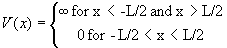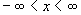# Please clearly state your assumptions, number the equations and indicate logical connections between different lines.

## 1.                  [2+2+2+2+2 pt] Infinite square well

Consider the potential.

a)      State all applicable boundary conditions.

b)      Find the time-dependent wavefunctions of the stationary states over the region -.

c)      Find the energy eigenvalues.

d)      For the ground state, evaluate Dx and Dp according to their definitions as rms deviation from the mean.  What is the product of Dx and Dp?

e)      For the first excited state, evaluate Dx and Dp according to their definitions as rms deviation from the mean.  What is the product of Dx and Dp?

## 2.                  [4 pt] Orthonormality of eigenfunctions of the infinite square well

Prove that the eigenfunctions of the infinite square well are orthonormal (eq. (35) on page 67).  Hint: You can write sin(ax) in as (eiax – e-iax)/(2i) and then do the integral.

## 3.                  [1+1+2+1+1pt] Particle in infinite square well

Ohanian, #3.5, p. 92